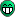# Accessing TTree values without Draw()

Dear ROOTers,

After reading a lot of examples and tutorials, I am using TTrees successfully now, but I couldn’t figure this one out:

I am actually looking for a function like Draw() but not for drawing, just for accessing the information, according to a selection pattern, e.g. a tree contains three branches containing x, y and f. Now it is possible to draw f vs y for a given x, using the Draw() function:

``t->Draw("f:y", "x==7")``

But how can I do exactly the same thing without using Draw() and without drawing. I just need the values, i.e. all the z and y values in the tree that match e.g. x==7, so I can loop only on those variables for further processingMaybe you are looking for the Scan method

Graphics output can be turned off with

``t->Draw("f:y", "x==7","goff")``

The htemp is created.

Hi,

thanks for quick reply. Turning off the graphics output is very nice. But the point is, of course Scan does also what I need, but like draw in an interactive way: it prints out the results on the screen for human interaction. I need to access the variables inside of a compiled program for looping.

So I am looking for a function that behaves like

``````t->Draw("f:y", "x==7")
or
t->Scan("f:y", "x==7")``````

but gives me back the entries for automatic analysis, not just print them on the screen. I am missing some derivation of GetEntry(“selection pattern”) which doesn’t seem to exist. So most probably this is intentional, and it can be done in a different way which I am not aware of.

any help is mostly appreciated.

cheers!thanks so far. Finally I found a workaround by looping through the whole tree several times, which worked and for the moment does the job.

``````Int_t nentries=(Int_t)t->GetEntries();
for (int j= 0; j < nentries; j++){
t->GetEntry(j);
if(x == 7)
do_something_with(f, y);
}``````

and I do it for every value of interest in x. I will update in case I found a more elegant way.

cheers.